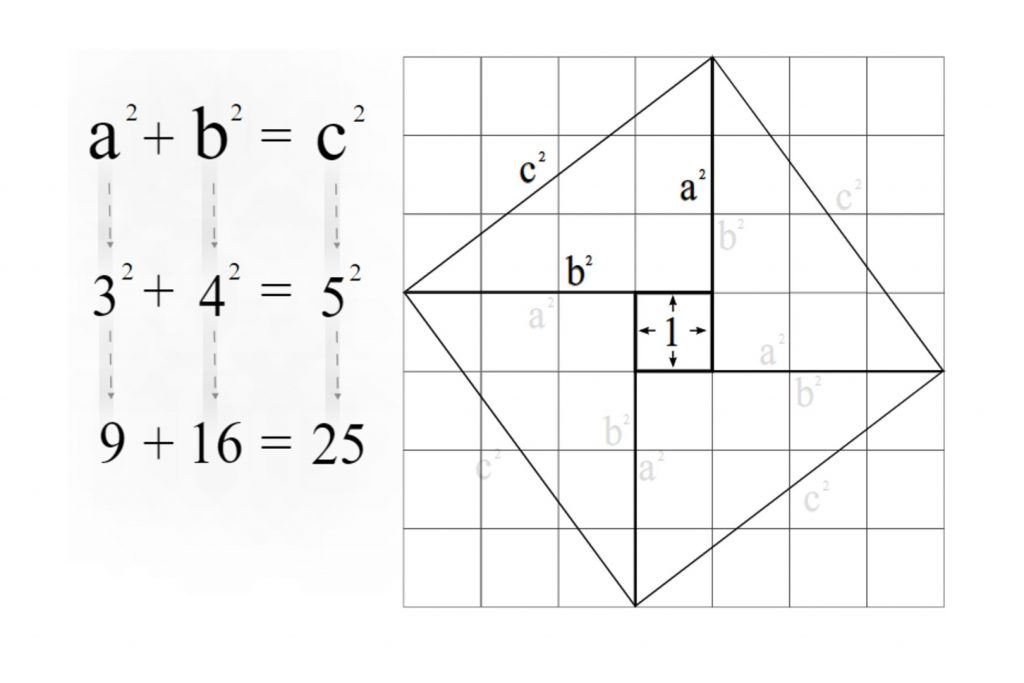## The Pythagorean Theorem

Right-angled triangle and the Pythagorean theorem In this article lets try to develop the Pythagorean theorem by means of the right angle. So first let’s get some proper ideas about the right-angled triangle. What is a right-angled triangle? If an angle of a triangle is 90o it is called a right-angled triangle or right triangle. …